Computational & Technology Resources an online resource for computational,engineering & technology publications not logged in - login Civil-Comp ProceedingsISSN 1759-3433 CCP: 77PROCEEDINGS OF THE NINTH INTERNATIONAL CONFERENCE ON CIVIL AND STRUCTURAL ENGINEERING COMPUTING Edited by: B.H.V. Topping Paper 11An Efficient Method for Decomposition of Regular Structures using Algebraic Graph Theory A. Kaveh and H. RahamiDepartment of Civil Engineering, Iran University of Science and Technology, Narmak, Tehran, Iran doi:10.4203/ccp.77.11 Full Bibliographic Reference for this paper A. Kaveh, H. Rahami, "An Efficient Method for Decomposition of Regular Structures using Algebraic Graph Theory", in B.H.V. Topping, (Editor), "Proceedings of the Ninth International Conference on Civil and Structural Engineering Computing", Civil-Comp Press, Stirlingshire, UK, Paper 11, 2003. doi:10.4203/ccp.77.11 Keywords: graphs, regular structures, strong Cartesian product, direct product,. Summary Algebraic graph theory can be considered as a branch of graph theory, where eigenvalues and eigenvectors of certain matrices are employed to deduce the principal properties of a graph. In fact eigenvalues are closely related to most of the invariants of a graph, linking one extremal property to another. These eigenvalues play a central role in our fundamental understanding of graphs. One of the major contributions in algebraic graph theory is due to Fiedler , where the properties of the second eigenvalue and eigenvector of the Laplacian of a graph have been introduced. This eigenvector, known as the Fiedler vector is used in graph nodal ordering and bipartition. General methods are available in literature for calculating the eigenvalues of matrices, however, for matrices corresponding to special models, it is beneficial to make use of their extra properties. In this paper an efficient method is presented for calculating the eigenvalues of regular structural models. A structure and correspondingly its graph model are called regular if they can be viewed as the direct or strong Cartesian product of some simple graphs known as their generators. The eigenvalues of the adjacency and Laplacian matrices for a regular graph model are easily obtained by the evaluation of eigenvalues of its generators. The second eigenvalue of the Laplacian of a graph is also obtained using a much faster and much simple approach than the existing methods Many structures have regular patterns and can be viewed as the strong Cartesian product or direct product of a number of simple graphs. These subgraphs used in the formation of a model are called the generators of that model. The Cartesian product of two graphs is used for nodal ordering and domain decomposition by Kaveh and Rahami [1,2]. In the following two other products are employed for graph partitioning and domain decomposition. The strong Cartesian product of the graphs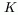and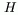, denoted by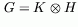has its node set as the Cartesian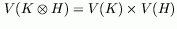and nodes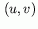and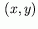of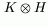being adjacent with respect to the strong product if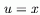and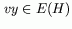, or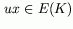and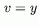, orand. For direct product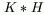the node set is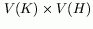. Two nodes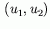and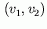are adjacent when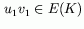and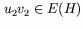. The following two theorems are used for calculating the eigenvalues for the Laplacian matrix of the products of two graphs: Theorem 1: Let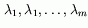and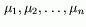be the eigenvalues of the adjacency matrices ofand, respectively. Then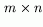eigenvalues ofare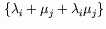for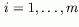and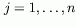. Theorem 2: Letandbe the eigenvalues of the adjacency matrices ofand, respectively. Theneigenvalues of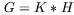are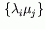forand. Formulae are derived for calculating the second eigenvalues of the Laplacian matrices. The second eigenvectors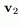are then obtained, and the bisection of the models are performed. This can be achieved by arranging the entries ofin an ascending order and partitioning the nodes into two subsets according to their occurrence in. References 1 M. Fiedler, Algebraic connectivity of graphs, Czechoslovak Mathematical Journal, 23(1973)298-305. 2 A. Kaveh and H. Rahami, A New Spectral Method for Nodal Ordering of Regular Space Structures, 2003, submitted for publication. doi:10.1016/j.finel.2003.11.007 3 A. Kaveh and H. Rahami, An efficient spectral method for bisection of regular finite element meshes, 2003, submitted for publication. purchase the full-text of this paper (price £20) Back to top ©Civil-Comp Limited 2023 - terms & conditions